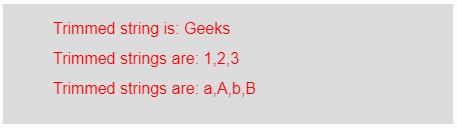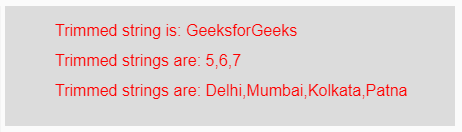# p5.js | trim() function

The trim() function in p5.js is used to remove whitespace characters and tab from the beginning and end of an input String. This function is also used to remove the carriage return and Unicode “nbsp” character.

Syntax:

`trim(Strings)`

or

`trim(Array_of_Strings)`

Parameters: This function accepts a parameter String or an array_of_strings which are going to be trimmed.

Return Value: It returns the trimmed Strings.

Below programs illustrate the trim() function in p5.js.
Example-1: This example uses trim() function to remove whitespace characters from the beginning and end of a String.

 `function` `setup() { ` ` `  `    ``// Creating Canvas size ` `    ``createCanvas(450, 120); ` `} ` ` `  `function` `draw() { ` ` `  `    ``// Set the background color  ` `    ``background(220); ` ` `  `    ``// Initializing the Strings and Array of strings ` `    ``let String = ``" Geeks "``; ` `    ``let Array1 = [``" 1 "``, ``" 2 "``, ``" 3 "``]; ` `    ``let Array2 = [``" a "``, ``" A "``, ``" b "``, ``" B "``]; ` ` `  `    ``// Calling to trim() function. ` `    ``let A = trim(String); ` `    ``let B = trim(Array1); ` `    ``let C = trim(Array2); ` ` `  `    ``// Set the size of text  ` `    ``textSize(16); ` ` `  `    ``// Set the text color  ` `    ``fill(color(``'red'``)); ` ` `  `    ``// Getting trimed strings ` `    ``text(``"Trimmed string is: "` `+ A, 50, 30); ` `    ``text(``"Trimmed strings are: "` `+ B, 50, 60); ` `    ``text(``"Trimmed strings are: "` `+ C, 50, 90); ` `} `

Output:Example-2: This example uses trim() function to remove tab from the beginning and end of a String.

 `function` `setup() { ` ` `  `    ``// Creating Canvas size ` `    ``createCanvas(450, 120); ` `} ` ` `  `function` `draw() { ` ` `  `    ``// Set the background color  ` `    ``background(220); ` ` `  `    ``// Initializing the Strings and Array of strings ` `    ``let String = ``"  GeeksforGeeks  "``; ` `    ``let Array1 = [``"  5  "``, ``"  6  "``, ``"  7  "``]; ` `    ``let Array2 = [``"  Delhi  "``, ` `                  ``"  Mumbai  "``, ` `                  ``"  Kolkata  "``, ` `                  ``"  Patna  "``]; ` ` `  `    ``// Calling to trim() function. ` `    ``let A = trim(String); ` `    ``let B = trim(Array1); ` `    ``let C = trim(Array2); ` ` `  `    ``// Set the size of text  ` `    ``textSize(16); ` ` `  `    ``// Set the text color  ` `    ``fill(color(``'red'``)); ` ` `  `    ``// Getting trimed strings ` `    ``text(``"Trimmed string is: "` `+ A, 50, 30); ` `    ``text(``"Trimmed strings are: "` `+ B, 50, 60); ` `    ``text(``"Trimmed strings are: "` `+ C, 50, 90); ` ` `  `} `

Output:Reference: https://p5js.org/reference/#/p5/trimMy Personal Notes arrow_drop_upCheck out this Author's contributed articles.

If you like GeeksforGeeks and would like to contribute, you can also write an article using contribute.geeksforgeeks.org or mail your article to contribute@geeksforgeeks.org. See your article appearing on the GeeksforGeeks main page and help other Geeks.

Please Improve this article if you find anything incorrect by clicking on the "Improve Article" button below.

Improved By : ManasChhabra2

Article Tags :

Be the First to upvote.

Please write to us at contribute@geeksforgeeks.org to report any issue with the above content.